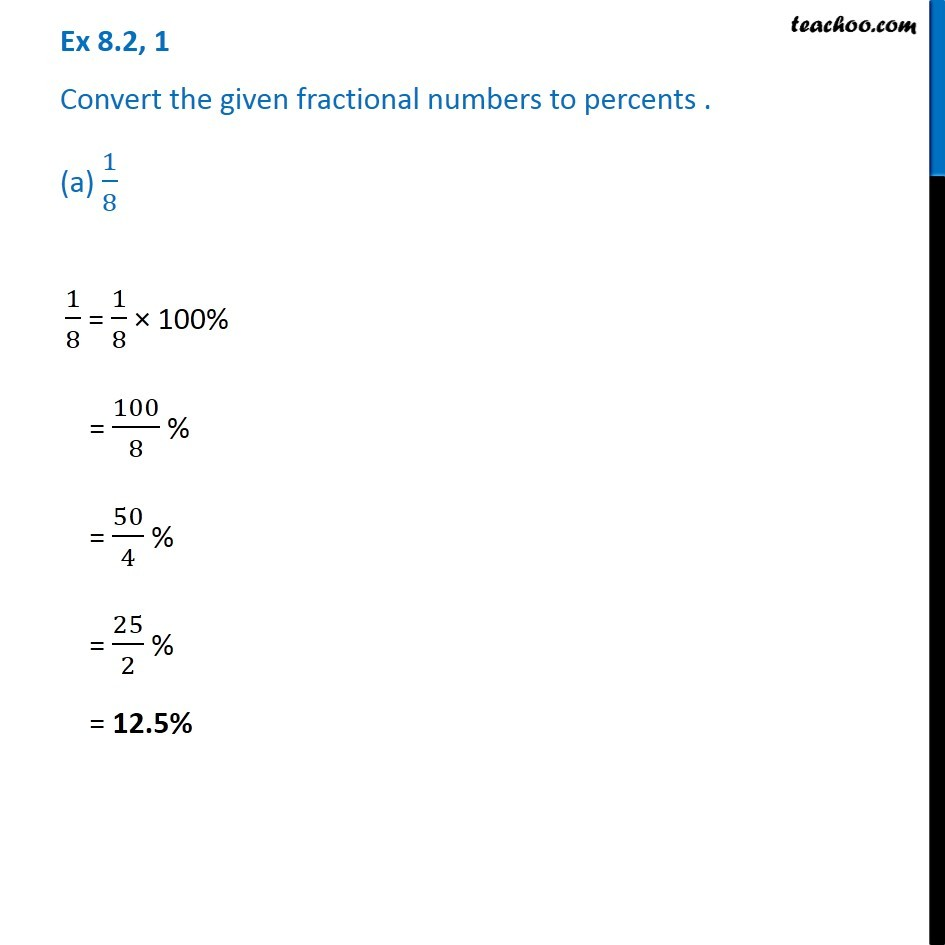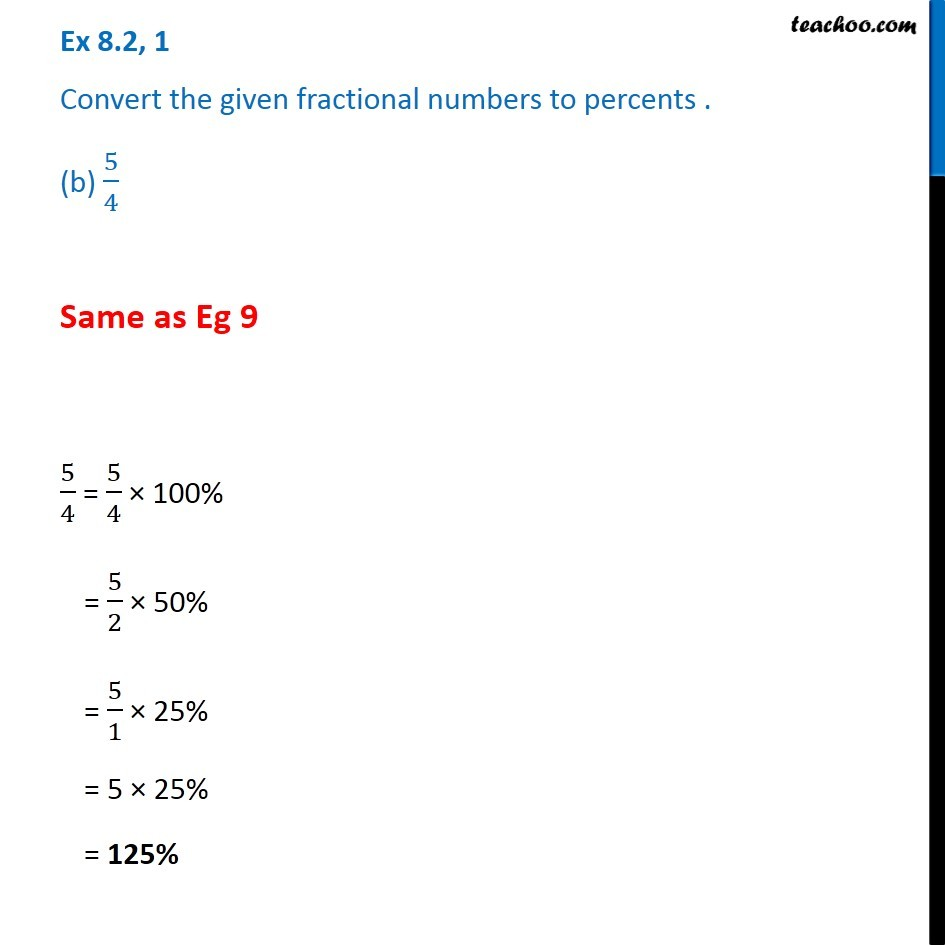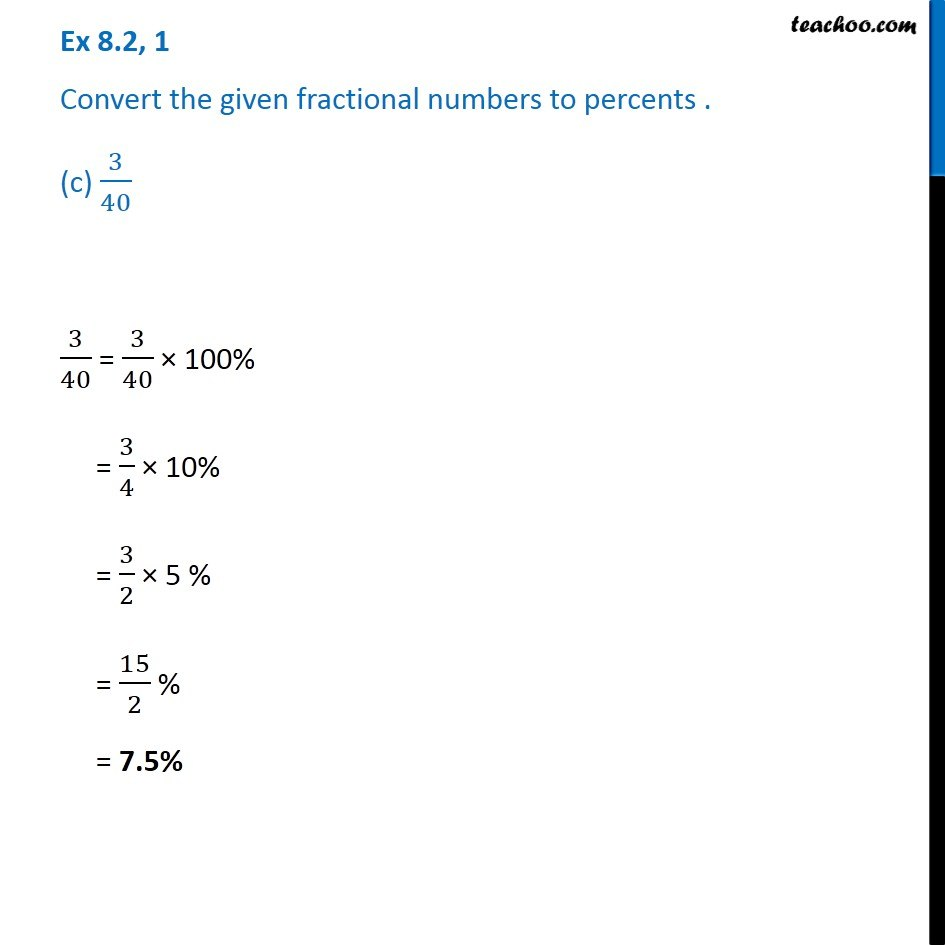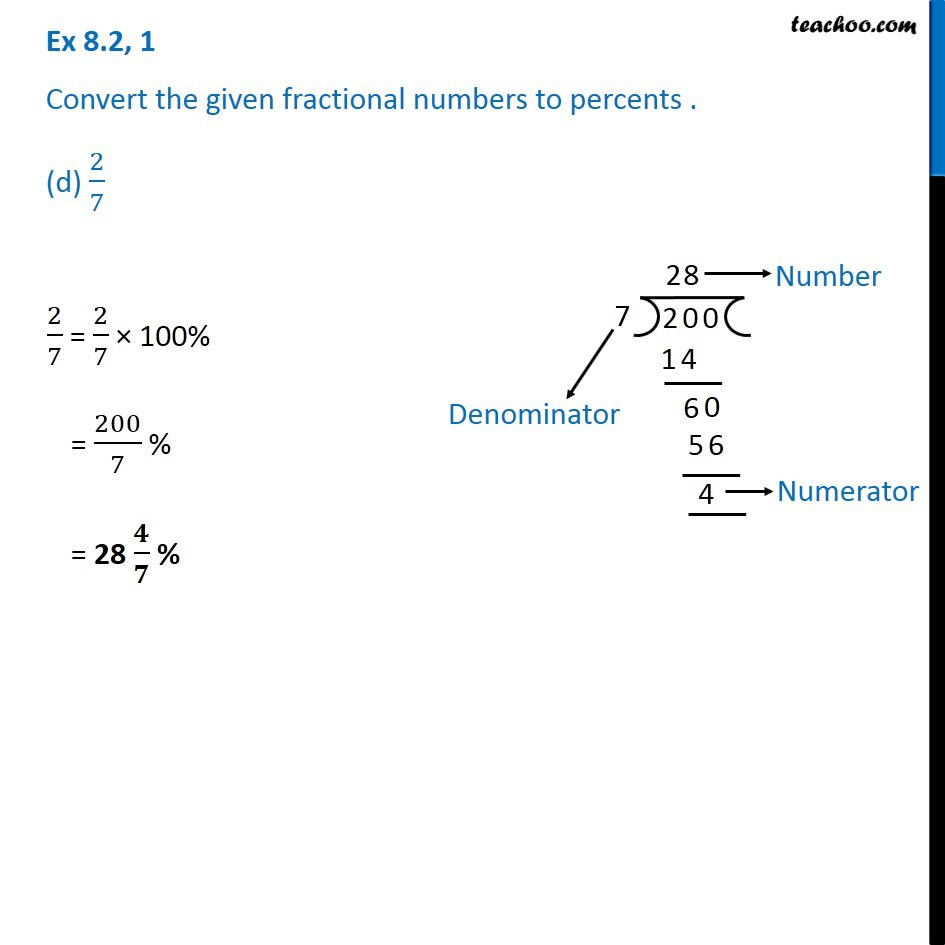1. Chapter 8 Class 7 Comparing Quantities
2. Serial order wise
3. Ex 8.2

Transcript

Ex 8.2, 1 Convert the given fractional numbers to percents . (a) 1/8 1/8 = 1/8 × 100% = 100/8 % = 50/4 % = 25/2 % = 12.5% Ex 8.2, 1 Convert the given fractional numbers to percents . (b) 5/4 Same as Eg 9 5/4 = 5/4 × 100% = 5/2 × 50% = 5/1 × 25% = 5 × 25% = 125% Ex 8.2, 1 Convert the given fractional numbers to percents . (c) 3/40 3/40 = 3/40 × 100% = 3/4 × 10% = 3/2 × 5 % = 15/2 % = 7.5% Ex 8.2, 1 Convert the given fractional numbers to percents . (d) 2/7 2/7 = 2/7 × 100% = 200/7 % = 28 𝟒/𝟕 %

Ex 8.2